Journal Details
Format
Journal
eISSN
1891-5469
First Published
01 Jan 2010
Publication timeframe
1 time per year
Languages
English
Open Access

# The effect of heart pulsatile on the measurement of artery bioimpedance

###### Page range:101 - 106
Journal Details
Format
Journal
eISSN
1891-5469
First Published
01 Jan 2010
Publication timeframe
1 time per year
Languages
English
Introduction

Bioimpedance is a widely used technique to measure the body composition due to its various advantages such as noninvasiveness, accuracy, applicability and low cost . The measurement of artery bioimpedance is proposed in this study because it is used to diagnose numerous of blood diseases such as the cholesterol level, the foundation of stenosis, and diabetes . Furthermore, the studying of the heart pulsatile effect may be used to measure the heart rate as a novel method for heart rate detection based on bioimpedance phenomena. The heart rate measuring using bioimpedance technique will be a new addition in medical devices unlike existing devices due to the already mentioned advantages .

A rated 17.5 million people died from cardiovascular disease (CVDs) in 2012, representing 31% of all global deaths. However, people who suffer from CVDs because of the existence of one or more risk reasons such as hypertension and diabetes, need early detection .

The electrical properties of biological tissues are divided into two categories corresponding to the source of the electrical signal, active and passive responses. Active response (bioelectricity) happens when biological tissue induces electricity because of ionic activities inside the cells. Passive response happens when biological tissues are stimulated by an external electrical current source [4, 5].

The heart pulsatile can be represented in finite element models (FEM) by two ways; first way is changing in blood pressure  and the second way is changing in the diameter of the artery wall .

The estimation of impedance depends on the electric current, which is passed through tissues. However, the electric current is described by two major parts (real and displacement), which are related to the generated electric field by the applied electrodes as follows :

$J=Jreal+JD=[σ(ω)+jωε(ω)]E,$$$J={{J}_{real}}+{{J}_{D}}=\left[ \sigma \left( \omega \right)+j\omega \varepsilon \left( \omega \right) \right]E,$$

where J: the total density of current, Jreal: the real part of current density, JD: the displacement part of current density, σ(ω): the conductivity of tissue dependent on frequency, ε(ω): the permittivity of tissue dependent on frequency. E: the generated electric field by the applied electrodes.

Equation (1) shows that the impedance depends on two parameters, as follows: 1) the generated electric field (which depends on the applied potential on electrodes, in addition to the position, the size and the shape of the electrodes) . 2) The dielectric properties of tissues (the conductivity and the permittivity).

The rest of this paper is arranged as follows: section 2 explains the steps of modeling of the artery bioimpedance measuring. Section 3 presents simulation results such as, the properties of applied electric field and the fluidic properties of blood. Finally, section 4 concludes this paper and summarizes how to simulate the effect of heart pulsatile on the measuring of artery bioimpedance.

2D Model of bioimpedance electrodes

COMSOL Multiphysics 5.0 is used for simulating the bioimpedance electrodes and an artery that is immersed in muscle and fat layers, and which are all covered by skin layer. The proposed model is simulated by three major stages: first stage is the pre-processing. Second stage is the processing stage as shown in Fig.1. In addition to the postprocessing stage in which the results are extracted and analyzed.

The pre-processing stage

As shown in Fig.1, the pre-processing stage contains: firstly, the selection of space dimensions, however, 2D space dimension is preferred to simplify the model in order to reduce the running time and increase the efficiency of the model. Secondly, the selection of physics, the electric current module is preferred to simulate the applied current and the generated electric field. The laminar flow module is selected to simulate and calculate the characteristics of blood motion based on its fluid dynamic properties. The frequency domain study is favored in order to compute the generated electric field at a specific range of frequencies. The laminar flow is used to simulate the motion of blood. The time-dependent study is preferred to compute the change in the motion of blood over time.

The processing stage

As shown in Fig.1, the processing stage contains: firstly, the geometry: which contains the structure of electrodes and composite layers (skin, fat, muscle, artery) as shown in Fig.2. The size and shape of electrode are proposed according to the results in article . The dimension of pulse in the geometry are estimated depend on the stroke volume in diastolic and systolic . Secondly, the materials: copper for electrodes, skin, fat and blood. Each material is described as set of features as shown in Table.1.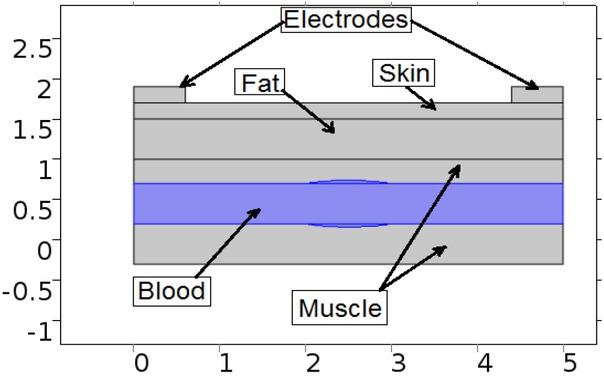The geometry of the electrodes and an artery immersed in muscle and fat layers and covered by a skin layer. All dimensions in cm .

The dielectric properties of organs at 100 kHz [10,12,13]. For blood as laminar flow: viscosity = 0.005 Pa∙s and density 1060 Kg/m3 .

OrganPermittivityConductivity (S/m)
1Skin10000.2
2Fat1000.4
3Muscle100000.4
4Blood40000.7
5Copper15.998e7

Thirdly, applied physics: laminar flow module; the blood pressure is defined by the following equations:

$Pin=126.09[mmHg]*f(t)$$${{\text{P}}_{\text{in}}}=126.09\left[ \text{mmHg} \right]*\text{f}\left( \text{t} \right)$$$Pout=125.415[mmHg]*f(t)$$${{\text{P}}_{\text{out}}}=125.415\left[ \text{mmHg} \right]*\text{f}\left( \text{t} \right)$$$f(t)=1−αcos(2π(t−0.5))0$$\text{f}\left( \text{t} \right)=1-\text{ }\!\!\alpha\!\!\text{ }\cos \left( 2\text{ }\!\!\pi\!\!\text{ }\left( \text{t}-0.5 \right) \right)0<\text{t}<1\,\,\,\,\,\,\,\,\left[ 14 \right]$$

where, Pin is the blood pressure at the inlet of the artery wall and Pout is the blood pressure at the outlet of the artery wall.

In addition to the electric current module, the properties of the applied electrical signal are 1mA, 1 kHz to 2 MHz, (current source) , and ground.

Finally, the mesh: whereas, the fine physics controlled mesh is proposed due to various advantages such as minimizing of the running time and increasing the efficiency of the model as shown in Fig.3., a physics controlled mesh which automatically creates meshes that are adapted to the physics in the model, can also be used.

The post-processing stage

In this stage, the results are extracted and analyzed for concluding the objective of the paper. The results extraction is illustrated in detail in the next section.

Results

In this part, the detailed results are described in three major categories as follows: first category, the applied electric field. Second category, the fluid mechanics of the blood. Third category, the bioimpedance.

The first category, the applied electric field is presented and described as shown in Fig.4. Fig.4 (A) shows the distribution of electric field over the whole layers. As noted in Fig.4 (A), the electric field intensity is higher at the skin and fat layer comparing to other layers because it is near to the electrodes. However, the legend bar show that the intensity of electric field at artery in suitable range to detect the changes in impedance after the losses due to the skin and fat layers. Fig.4 (B) shows that the path of the electric field starts in one electrode and continues to the second electrode through the artery, which confirms the ability of the system to measure the impedance of the artery with sufficient efficiency. Fig.4 (C) shows the intensity of the electric field with respect to the length of the artery as shown in Fig.4 (D). However, the intensity of the electric field is increased around the pulse.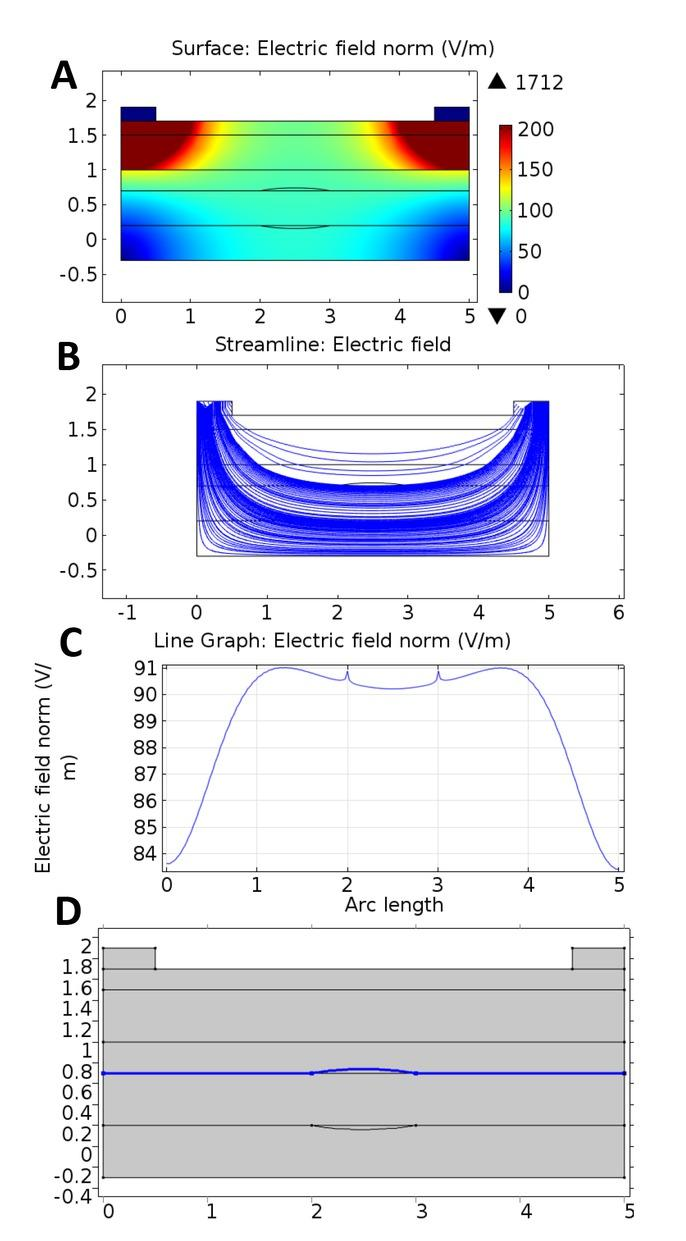The properties of the applied electric field: A) the distribution of electric field over all layers, B) the stream of electric field through all layers, C) the distribution of electric field along the artery, and D) the arc length of (C).

Fig.5 shows the fluid dynamic properties of blood. The translation velocity of blood is described in Fig.5 (A), which is estimated according to the following equations: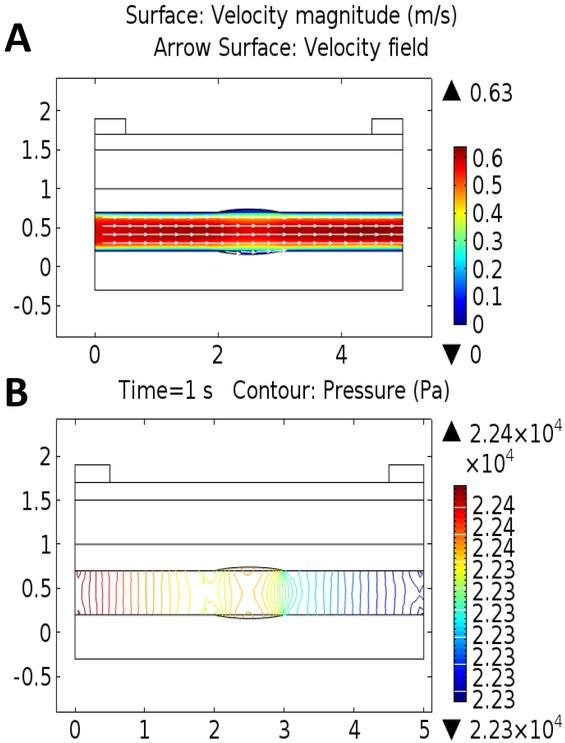The dynamic fluid properties of blood: A) the velocity, and B) the pressure.$ρ∂u∂t+ρ(u⋅∇)u=∇⋅[−p+μ(∇u+(∇u)T −23μ(∇⋅u)I)]+F$\begin{align}& \rho \frac{\partial u}{\partial t}+\rho \left( u\cdot \nabla \right)u=\nabla \cdot \left[ -p+\mu \left( \nabla u+{{\left( \nabla u \right)}^{T}} \right. \right. \\ & \,\,\,\,\,\,\,\,\,\,\,\,\,\,\,\,\,\,\,\,\,\,\,\,\,\,\,\,\,\,\,\,\,\,\,\,\,\,\,\,\,\,\,\,\,\,\,\,\,\,\,\,\,\,\,\,\,\,\left. \,\left. -\frac{2}{3}\mu \left( \nabla \cdot u \right)I \right) \right]+F \\ \end{align}$∂ρ∂t+∇⋅(ρμ)=0$$$\frac{\partial \rho }{\partial t}+\nabla \cdot \left( \rho \mu \right)=0$$

where u is the fluid velocity, p is the fluid pressure, ρ is the fluid density, and μ is the fluid dynamic viscosity.

Eq.5 is divided into four major parts as follows: First part is the inertial forces at the left hand side. The second part is the pressure forces which is represented by the part (−p ∙ I). Third part is the viscous forces, which is represented by the part $(μ(∇u+(∇u)T−23μ(∇⋅u)I)).$$\left( \mu \left( \nabla u+{{\left( \nabla u \right)}^{T}}-\frac{2}{3}\mu \left( \nabla \cdot u \right)I \right) \right).$ Finally, F represents the external applied force to the fluid. However, the important parts in this study are the pressure forces (the pressure during systolic and diastolic) and viscous forces (the viscosity of the blood).

Eq.5 is always solved together with the continuity equation eq.6. However, Eq.5 represents the conservation of momentum, while the continuity equation (Eq.6) represents the conservation of mass. Furthermore, Fig.5 (B) describes the distribution of blood pressure along the artery Eq. (2, 3, 4) .

Discussion

The effect of inserting a pulse, which is an extension in the diameter of the artery due to the stroke volume, on the measured artery impedance is presented in the Fig.6. Where, the blue line represents the impedance of the artery and all layers versus different frequencies in case of artery wall extension. The red line represents the impedance of artery and all layers versus different frequencies in case of inserting a pulse extension in the diameter of the artery. The difference between the two lines represent the errors in the calculations for any application, therefore, it is very important to track the heart pulsatile effect in the model conditions for different applications. Furthermore, the heart rate can be estimated from this difference by counting the times the impedance is changed from blue line to red line for one minute. From this point, a novel method for measuring heart rate based on bioimpedance is presented.

Fig.7 shows the effect of changing the blood pressure by retyping the equations (2,3) as follows: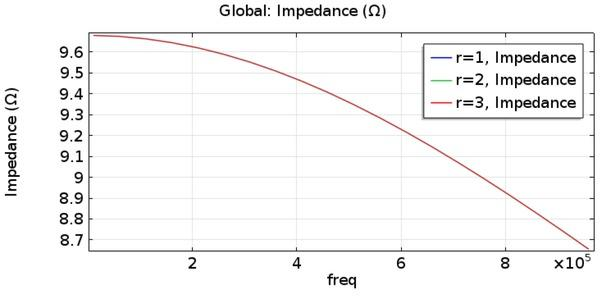The magnitude of the impedance of all layers versus range of frequencies at different values of blood pressures.$Pin=r⋅126.09[mmHg]⋅f(t)$$$\text{Pin}=\text{r}\cdot 126.09\left[ \text{mmHg} \right]\cdot \text{f}\left( \text{t} \right)$$$Pout=r⋅125.415[mmHg]⋅f(t)$$${{\text{Pout}}}=\text{r}\cdot 125.415\left[ \text{mmHg} \right]\cdot \text{f}\left( \text{t} \right)$$

where, r is a factor for comparing impedance curve with respect to frequency at different inlet and outlet pressures.

Note that all lines are plotted on each other, which mean that the changing in pressure has no effect on the measured impedance. Fig.6 shows that neglecting the effect of the heart pulsatile cannot be considered as an accurate model, because there will be a certain error in the results due to the difference between the lines.

Fig.7 confirms the conclusion in article , which is the effect of pressure on the dielectric properties of tissue (conductivity) do not appear apart from at very high pressure (GPa). Therefore, the results show that the variation in pressure is not able to represent the heart pulsatile effect in the FEM model.

In the following, we will use glucose level measurement by bioimpedance technique to show the effect of neglecting the effect of the heart pulsatile on the results. The relationship between the dielectric properties of blood and the glucose concentration in blood is presented in Fig.8 .

As shown in Fig.8 (A), the permittivity of the blood gradually decreases with increasing concentration of glucose in blood. In addition, the conductivity of the blood is also decreasing with increasing concentration of glucose in blood as shown in Fig.8 (B). This relationship between the dielectric properties and the glucose concentration in blood leads to measuring the glucose level based on the changes in the bioimpedance as shown in Fig.9.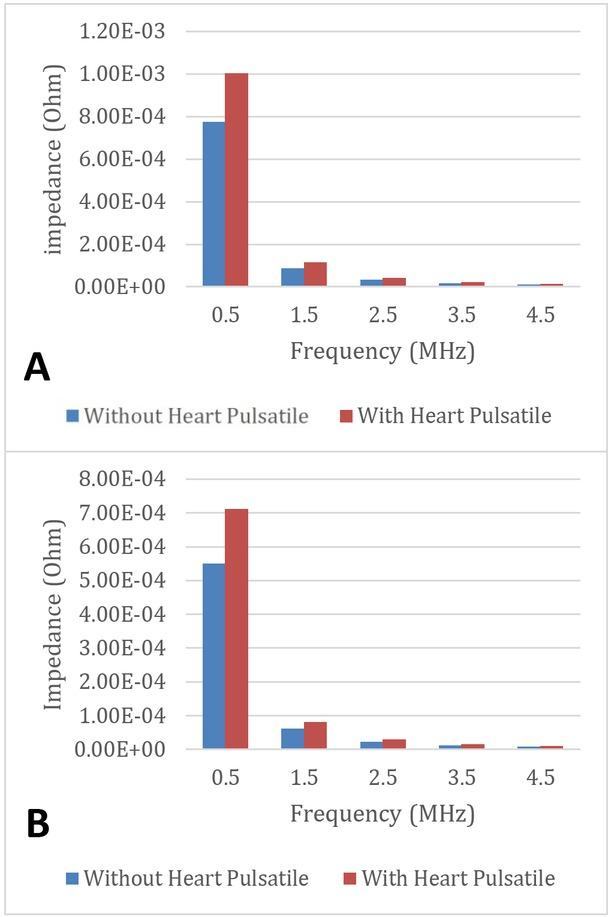Comparison between measuring the glucose level with and without heart pulsatile at different level of glucose concentration: A) 0 mg/dl, and B) 2000 mg/dl.

The effect of ignoring the heart pulsatile effect on the measurement at different blood glucose concentrations is described in Fig.9. Fig.9 shows that the values of impedance at different blood glucose concentrations (0 and 2000 mg/dl) without the pulsatile effect are much less than with the pulsatile effect at the first part of frequency range. However, Fig.9 shows that there are errors in the expectation of glucose level using the magnitude of the measured impedance as described at the 0.5 MHz point in both cases. Where, at 0.5 MHz, and 0 mg/dl glucose concentration; the difference between two bars (without and with the pulsatile effect) is 2.279×10−4 ohm and 1.613×10−4 ohm at 2000 mg/dl glucose concentration, which represent the error in the measurement of glucose level in case of neglecting the heart pulsatile effect.

Therefore, Fig.9 confirms the objective of this article, which is the importance of adding the heart pulsatile effect to the estimation of blood diseases based on the bioimpedance technique.

Conclusion

A model of non-invasive electrodes for measurement of artery bioimpedance and a model of composite layers is presented and discussed. In addition, a model of blood glucose level detection is discussed.

The simulation results show that the best method of taking into consideration the effect of heart pulsatile is the extension in the diameter of artery wall as a pulse. Furthermore, the results show that an error exists in the calculations of glucose level detection using bioimpedance electrodes in case of neglecting the effect of the heart pulsatile effect.

#### The dielectric properties of organs at 100 kHz [10,12,13]. For blood as laminar flow: viscosity = 0.005 Pa∙s and density 1060 Kg/m3 .

OrganPermittivityConductivity (S/m)
1Skin10000.2
2Fat1000.4
3Muscle100000.4
4Blood40000.7
5Copper15.998e7

Khalil, S.F., Mohktar, M.S. and Ibrahim, F., 2014. The theory and fundamentals of bioimpedance analysis in clinical status monitoring and diagnosis of diseases. Sensors, 14(6), pp.10895-10928. https://doi.org/10.3390/s140610895KhalilS.F.MohktarM.S.IbrahimF.2014The theory and fundamentals of bioimpedance analysis in clinical status monitoring and diagnosis of diseasesSensors1461089510928doi.org/10.3390/s14061089510.3390/s140610895411836224949644Search in Google Scholar

Bera, T.K., 2014. Bioelectrical impedance methods for noninvasive health monitoring: a review. Journal of Medical Engineering, 2014. https://doi.org/10.1155/2014/381251BeraT.K.2014Bioelectrical impedance methods for noninvasive health monitoring: a reviewJournal of Medical Engineering2014doi.org/10.1155/2014/38125110.1155/2014/381251478269127006932Search in Google Scholar

Kyle U.G., Bosaeus I., De Lorenzo A.D., Deurenberg P., Elia M., Gómez J.M., Heitmann B.L., Kent-Smith L., Melchior J.-C., Pirlich M. Bioelectrical impedance analysis—Part i: Review of principles and methods. Clin. Nutr. 2004;23:1226–1243. https://doi.org/10.1016/j.clnu.2004.06.004KyleU.G.BosaeusI.DeLorenzo A.D.DeurenbergP.EliaM.GómezJ.M.HeitmannB.L.Kent-SmithL.MelchiorJ.-C.PirlichM.Bioelectrical impedance analysis—Part i: Review of principles and methodsClin. Nutr20042312261243doi.org/10.1016/j.clnu.2004.06.004

Martinsen Ø.G., Grimnes S. Bioimpedance and Bioelectricity Basics. Academic Press; Waltham, MA, USA: 2010.MartinsenØ.G.GrimnesSBioimpedance and Bioelectricity BasicsAcademic PressWaltham, MA, USA2010Search in Google Scholar

Mitchell, A.C. and Nellis, W.J., 1982. Equation of state and electrical conductivity of water and ammonia shocked to the 100 GPa (1 Mbar) pressure range. The Journal of Chemical Physics, 76(12), pp.6273-6281. https://doi.org/10.1063/1.443030MitchellA.C.NellisW.J.1982Equation of state and electrical conductivity of water and ammonia shocked to the 100 GPa (1 Mbar) pressure rangeThe Journal of Chemical Physics761262736281doi.org/10.1063/1.443030

Dai, T. and Adler, A., 2006, May. Blood impedance characterization from pulsatile measurements. In 2006 Canadian Conference on Electrical and Computer Engineering (pp. 983-986). IEEE. https://doi.org/10.1109/CCECE.2006.277500DaiT.AdlerA.2006Blood impedance characterization from pulsatile measurements2006 Canadian Conference on Electrical and Computer Engineering983986IEEEdoi.org/10.1109/CCECE.2006.277500

Stiles, D.K. and Oakley, B.A., 2003, September. Comparison of conformal and nonconformal meshes in the electromagnetic simulation of atherosclerotic lesions. In Engineering in Medicine and Biology Society, 2003. Proceedings of the 25th Annual International Conference of the IEEE (Vol. 4, pp. 3005-3008). IEEE. https://doi.org/10.1109/IEMBS.2003.1280772StilesD.K.OakleyB.A.2003Comparison of conformal and nonconformal meshes in the electromagnetic simulation of atherosclerotic lesionsEngineering in Medicine and Biology Society, 2003Proceedings of the 25th Annual International Conference of the IEEEVol. 430053008IEEEdoi.org/10.1109/IEMBS.2003.1280772

Christophe Rubeck , Stéphane BONNET , Fadwa Ben Amara , Claire Pereira , Sadok GHARBI. 2015. A human skin model for body hydration monitoring by electrical impedance measurement. COMSOL conference.ChristopheRubeckStéphaneBONNETFadwaBen AmaraClairePereiraSadokGHARBI.2015A human skin model for body hydration monitoring by electrical impedance measurementCOMSOL conferenceSearch in Google Scholar

Noisakran, S., Onlamoon, N., Songprakhon, P., Hsiao, H.M., Chokephaibulkit, K. and Perng, G.C., 2010. Cells in dengue virus infection in vivo. Advances in virology, 2010. https://doi.org/10.1155/2010/164878NoisakranS.OnlamoonN.SongprakhonP.HsiaoH.M.ChokephaibulkitK.PerngG.C.2010Cells in dengue virus infection in vivoAdvances in virology, 2010doi.org/10.1155/2010/16487810.1155/2010/164878327605722331984Search in Google Scholar

Nakatani, S., Yamagishi, M., Tamai, J., Goto, Y., Umeno, T., Kawaguchi, A., Yutani, C. and Miyatake, K., 1995. Assessment of coronary artery distensibility by intravascular ultrasound. Circulation, 91(12), pp.2904-2910. https://doi.org/10.1161/01.CIR.91.12.2904NakataniS.YamagishiM.TamaiJ.GotoY.UmenoT.KawaguchiA.YutaniC.MiyatakeK.1995Assessment of coronary artery distensibility by intravascular ultrasoundCirculation911229042910doi.org/10.1161/01.CIR.91.12.2904

Stiles, D.K. and Oakley, B.A., 2003, September. Comparison of conformal and nonconformal meshes in the electromagnetic simulation of atherosclerotic lesions. In Engineering in Medicine and Biology Society, 2003. Proceedings of the 25th Annual International Conference of the IEEE (Vol. 4, pp. 3005-3008). IEEE. https://doi.org/10.1109/IEMBS.2003.1280772StilesD.K.OakleyB.A.2003 Comparison of conformal and nonconformal meshes in the electromagnetic simulation of atherosclerotic lesionsEngineering in Medicine and Biology Society, 2003Vol. 430053008IEEEdoi.org/10.1109/IEMBS.2003.1280772

Ferreira, D.W. and Lebensztajn, L., 2013. A systematic sensitivity analysis of the performance of a transcutaneous energy transmitter for design purposes. Journal of Microwaves, Optoelectronics and Electromagnetic Applications, 12(2), pp.292-306. https://doi.org/10.1590/S2179-10742013000200005FerreiraD.W.LebensztajnL.2013A systematic sensitivity analysis of the performance of a transcutaneous energy transmitter for design purposesJournal of Microwaves, Optoelectronics and Electromagnetic Applications122292306doi.org/10.1590/S2179-1074201300020000510.1590/S2179-10742013000200005Search in Google Scholar

Fluid-structure interaction in a network of blood vessels, model, COMSOL.Fluid-structure interaction in a network of blood vessels, modelCOMSOLSearch in Google Scholar

Anand, G., Lowe, A. and Al-Jumaily, A.M., 2016. Simulation of impedance measurements at human forearm within 1 kHz to 2 MHz. Journal of Electrical Bioimpedance, 7(1), pp.20-27. https://doi.org/10.5617/jeb.2657AnandG.LoweA.Al-JumailyA.M.2016Simulation of impedance measurements at human forearm within 1 kHz to 2 MHzJournal of Electrical Bioimpedance712027doi.org/10.5617/jeb.2657

Mitchell, A.C. and Nellis, W.J., 1982. Equation of state and electrical conductivity of water and ammonia shocked to the 100 GPa (1 Mbar) pressure range. The Journal of Chemical Physics, 76(12), pp.6273-6281. https://doi.org/10.1063/1.443030MitchellA.C.NellisW.J.1982Equation of state and electrical conductivity of water and ammonia shocked to the 100 GPa (1 Mbar) pressure rangeThe Journal of Chemical Physics761262736281doi.org/10.1063/1.443030

Topsakal, E., Karacolak, T. and Moreland, E.C., 2011, August. Glucose-dependent dielectric properties of blood plasma. In General Assembly and Scientific Symposium, 2011 XXXth URSI (pp. 1-4). IEEE. https://doi.org/10.1109/URSIGASS.2011.6051324TopsakalE.KaracolakT.MorelandE.C.2011Glucose-dependent dielectric properties of blood plasmaGeneral Assembly and Scientific Symposium, 2011 XXXth URSI14doi.org/10.1109/URSIGASS.2011.6051324

Recommended articles from Trend MD